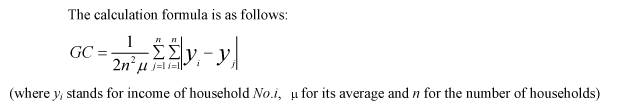Appended Note 3-4 The Gini coefficient

This is an indicator which shows by means of a numeric value how income distribution has become lopsided compared to the case where the income has been distributed in a perfectly equal way. If it is perfectly equal, the value is zero, and if it is perfectly unequal (the entire society's income is possessed exclusively by one person and the others' income is zero), the value is one. Geometrically, this Gini coefficient is expressed as a ratio of A to B, where A stands for the area of a crescent-shaped portion surrounded by the "Lorenz curve" which is obtained by plotting the accumulative household ratio and the accumulative income ratio, and the diagonal, and B stands for the area of a right-angle isosceles triangle with the diagonal as its oblique line. The more equally income is distributed, the closer the Lorenz curve is to the diagonal, and the more unequally income is distributed, the more the curve bends downward. It is relatively sensitive to the change in the income distribution among middle-income groups.There are cases where samples with a minus value are included when Gini coefficients such as household assets are prepared. Conceptually, the maximum of the Gini coefficient may possibly be larger than one.

Note: 1. Reference: "Social Security Reforms in an Era with Dwindling Population" (by T. Oshio, 2005)